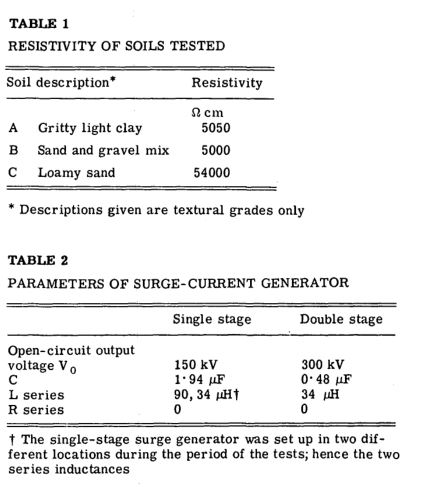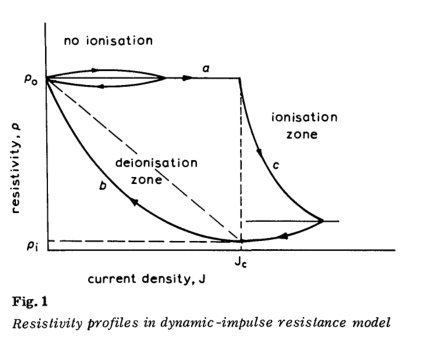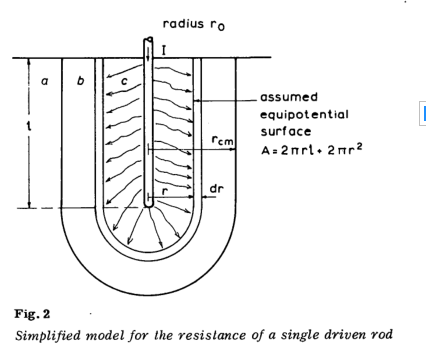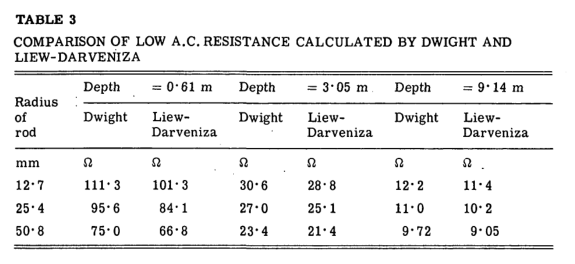# Dynamic model of impulse characteristics of concentrated earths

ps：所有的从动杆或驱动杆的意思都是接地极

## 符号说明

$R_E$接地电阻 $V$地电阻电压 $I$入地电流 $\rho$土壤电阻率 Ωcm $\rho_0$低电流下的土壤电阻率 Ωcm $g_c$击穿电压梯度 kV/cm $J$电流密度 $kA/cm^{-2}$ $J_c$击穿电流密度 $kA/cm^{-2}$ $r$单元半径 $r_{cm}$最大临界半径 $r_c$临界击穿半径 $d_r$外壳厚度 $r_0$从动杆半径 $l$从动杆深度 $d$从动杆之间的分离 $R_s$电泳发生器的串联电阻 $L_s$电泳发生器的串联电抗 $C$电泳发生器的电容 $V_0$电泳发生器的输出开路电压 $B$电流密度能力指数 $\tau_1$电离时间常数 $\tau_2$去电离时间常数

## 2. 实验

（a）将直径12’7毫米的杆驱动0. 61 m（2ft）进入土壤 （b）将直径25’4毫米的杆驱动0.61 m（2ft）进入土壤（使用土壤A时，将电极驱动1.83 m） （c）埋在土壤表面的直径152.4 mm的半球形电极 （d）产生火花连接; 通过放置在土壤表面上方的直径为12 * 7毫米的杆进行模拟。（a）非线性电压/电流特性 （b）电压波的峰值出现在电流峰值之前 （c）最小电阻的瞬间出现在电流峰值之后 （d）在电阻/电流中观察到明显的磁滞效应 （e）当电流降至零时，可以看到特性曲线。

## 3. 提出动态模型

### 3.1 动态模型中的基本假设

（a）土壤是均质的和各向同性的； 即在所有方向上的电阻率都相同。

（b）随着电流累积并渗透到土壤中，电流密度大于临界值的区域的电阻率将小于低电流值$\rho_0$。 这对应于击穿，并假定是由于电离增加而随时间变化的扩散生长而发生的。 电阻率从$\rho_0$的较高值到$\rho$的较低状态应具有随时间变化或衰减的时间依赖性

$\rho = \rho _ { 0 } \operatorname { exp } \frac { - t } { \tau _ { 1 } }$

（c）随着电流的减小，电阻率应以与时间呈指数关系并且与电流密度成指数关系的方式恢复到其原始值$\rho_0$。 前者是一个名词

$( 1 - \operatorname { exp } \frac { - t } { \tau _ { 2 } } )$

$( 1 - \frac { J } { J _ { C } } ) ^ { B }$### 3.2 单从动杆电阻的计算

#### 3.2.1 单根驱动杆的交流线性电阻$dR = \frac { \rho d r } { 2 \pi ( r ^ { 2 } + r \ell ) } = \frac { \rho dr } { 2 \pi \ell } ( \frac { 1 } { r } - \frac { 1 } { r + \ell } )$

$\left. \begin{array}{l}{ R = \int _ { r _ { 0 } } ^ { \infty } \frac { \rho } { 2 \pi \ell } ( \frac { 1 } { r } - \frac { 1 } { r + \ell } ) d }\{ = \frac { \rho } { 2 \pi \ell } ( \operatorname { ln } \frac { r + \ell } { r } ) _ { \infty } ^ { r _ { 0 } } }\end{array} \right.$

$R = \frac { \rho } { 2 \pi \ell } \operatorname { ln } \frac { r _ { 0 } + \ell } { r _ { 0 } }\tag{1}$Dwight 方程：

$R = \frac { \rho } { 2 \pi \ell } ( \operatorname { ln } \frac { 4 \ell } { r _ { 0 } } - 1 )$

Liew-Darveniza方程：

$R = \frac { \rho } { 2 \pi \ell } \operatorname { ln } \frac { r _ { 0 } + \ell } { r _ { 0 } }$

#### 3.2.2 单从动杆的抗冲击性

$J = \frac { I } { 2 \pi r ^ { 2 } }\tag{2}$

$\text{Voltage across element} = \frac { \text { I } \rho dr } { 2 \pi r ^ { 2 } } \tag{3}$

$\frac { I \rho _ { 0 } dr } { 2 \pi r ^ { 2 } } > g _ { c } dr \tag{4}$

$J _ { c } = \frac { g _ { c } } { \rho _ { 0 } }\tag{5}$

（a）电阻率在$\rho_0$处保持恒定，直到元件的电流密度超过临界值为止

$\rho = \rho _ { 0 } \text { for } J < J _ { C }$

（b）对于超过临界值的电流密度，电阻率以指数方式衰减，对应于土壤中的电离

$\rho = \rho _ { 0 } \operatorname { exp } \frac { - t } { \tau _ { 1 } } \text { for } J \geq J _ { C }$

（i）区域a，其中$r > r_{c m}$和$J < J_c$。 该区域对应于没有被完全电离。 因此

$\rho = \rho _ { 0 } \tag{6}$

（ii）b区，其中$r < r_{c m}$和$J < J_c$。 现在，电流密度J低于临界值，土壤电阻率通过以下方式恢复：

$\rho = \rho _ { i } + ( \sigma _ { 0 } - \rho _ { i } ) ( 1 - \operatorname { exp } \frac { - t } { \tau _ { 2 } } ) ( 1 - \frac { J } { J _ { C } } ) ^ { 2 } \tag{7}$

（iii）c区域，其中$r < r_{c m}$和$J \geq J_c$。 电流密度J仍超过临界值，并且电离继续：

$\rho = \rho _ { 0 } \operatorname { exp } \frac { - t } { \tau _ { 1 } } \tag{8}$